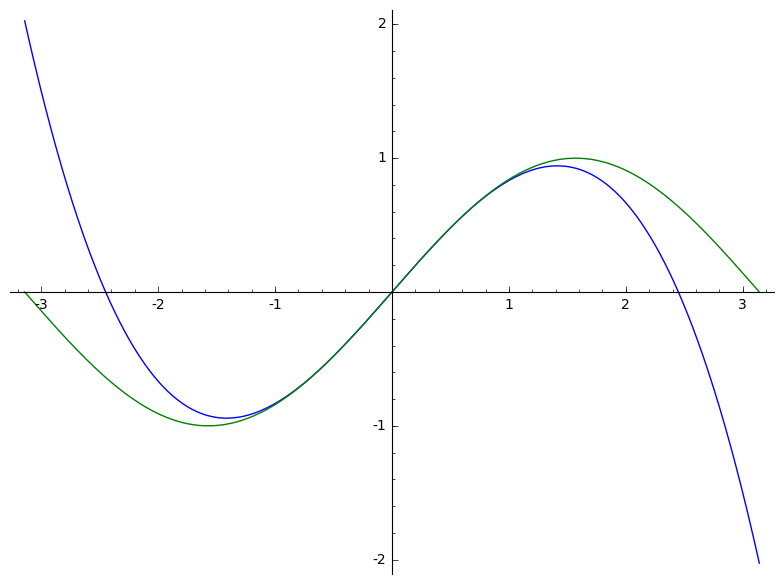# Polynomials in sagemath¶

Some useful global variables:

Natural Numbers (semiring) Integer Ring ℕ NN ℤ ZZ ℚ QQ ℝ RR ℂ CC

At first we create a polynomial ring over a field/ring

In :
R.<x> = PolynomialRing(QQ)
R.<x> = QQ[]


Also a multivariate polynomial ring is possible

In :
R.<x,y> = PolynomialRing(QQ)


Another useful way to get x

In :
x = PolynomialRing(QQ, 'x').gen()


Now we can declare some polynomial:

In :
p = x^3 + 0.3*x^2 - x - 0.3
p

Out:
x^3 + 0.300000000000000*x^2 - x - 0.300000000000000

How to evaluate the value at some given point a?

In :
p(-0.1)

Out:
-0.198000000000000

We can also factor p

In :
p.factor()

Out:
(x - 1.00000000000000) * (x + 0.300000000000000) * (x + 1.00000000000000)

At next we calculate the roots

In :
p.roots()

Out:
[(-1.00000000000000, 1), (-0.300000000000000, 1), (1.00000000000000, 1)]

A short introduction into plotting polynomials

In :
plot(p)

Out: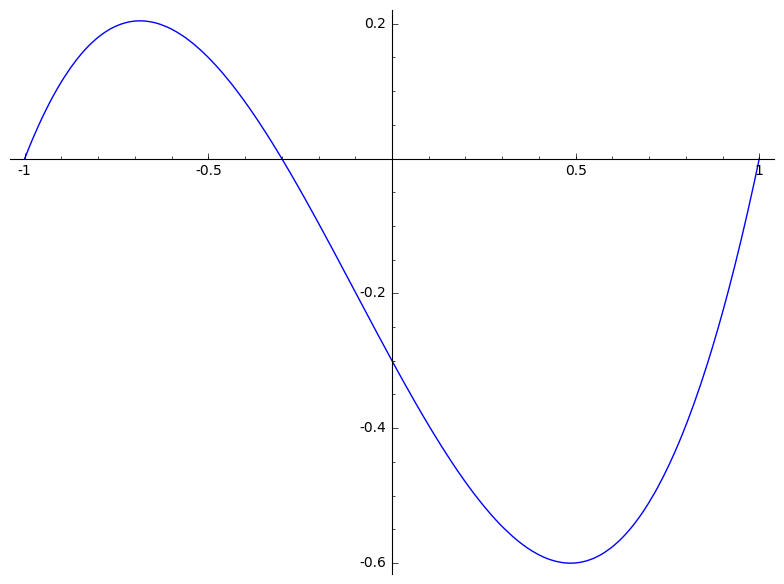In this example, the domain is chosen by sagemath. In the following, we supply minimum and maximum x-value explicitly:

In :
var('s')
plot(p(s), (s, -5, 5))

Out: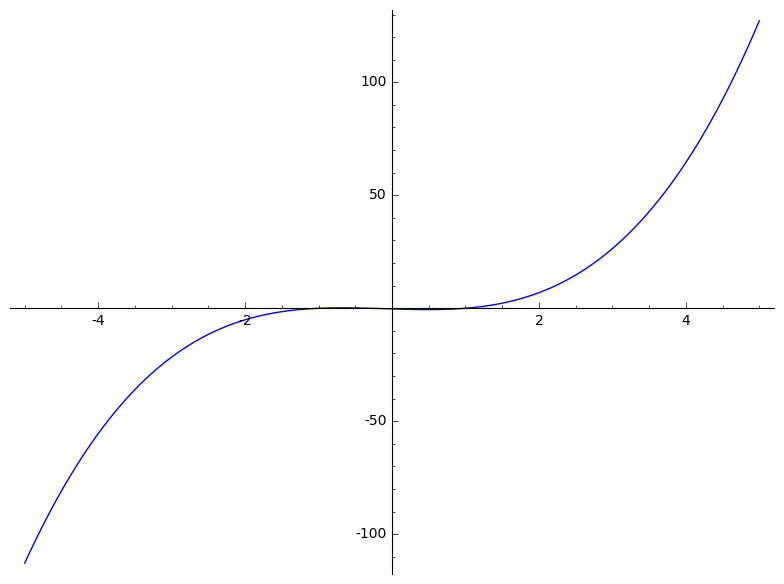Calculate the derivative

In :
p_diff = p.diff()
p_diff

Out:
3.00000000000000*x^2 + 0.600000000000000*x - 1.00000000000000

Combine plots and zoom in

In :
plot(p(s), (s, -10, 10)) + plot(p_diff(s), (s, -10, 10), color='red')

Out: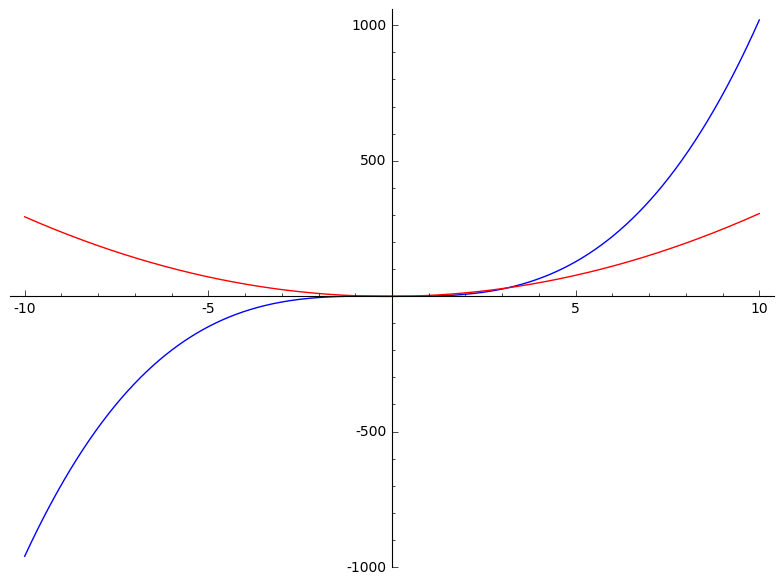Calculate the integral

In :
p_int = p.integral()
p_int

Out:
0.250000000000000*x^4 + 0.100000000000000*x^3 - 0.500000000000000*x^2 - 0.300000000000000*x

Plot the integral and the polynomial

In :
plot(p(s), (s, -10, 10)) + plot(p_int(s), (s, -10, 10), color='green')

Out: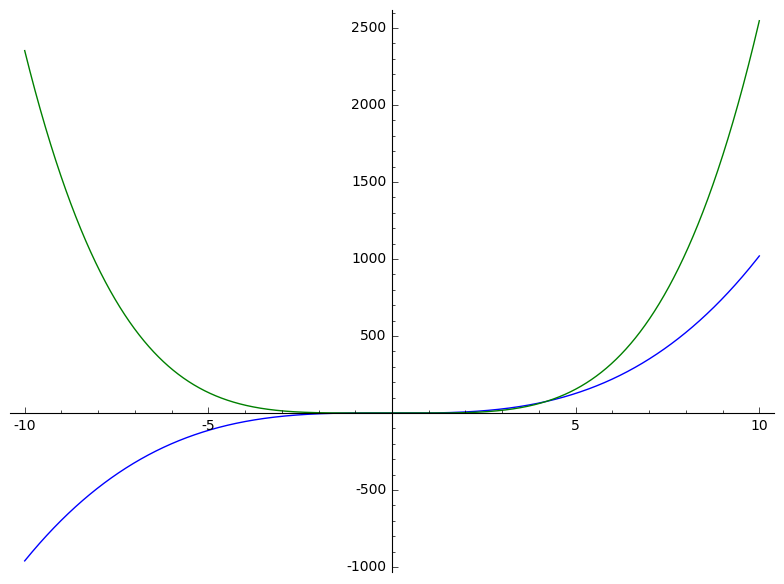One particular case of a polynomial is a power series. Sine is a trigonometric function defined as a power series:

In :
x = var('x')
f = sin(x)
s = f.series(x, 5)
show(s)
show(s(x=5))


Now we can draw the sine and the polynomial to see the differences

In :
plot(s.truncate(), xmin=-pi, xmax=pi) + plot(f, color='green', xmin=-pi, xmax=pi)

Out: